Windmill DIY Analog MPPT Circuit

Author Message
bobshauNewbie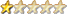Joined: 22/11/2009
Location: United States
Posts: 27
 Posted: 02:29pm 26 Nov 2009

Windmill DIY Analog MPPT Circuit Design and Construction

Purpose:
I would like to share the design of my Windmill maximum power point tracking (MPPT) circuit in enough detail so someone with some electronics experience could make it for their windmill. I am pleased with the performance, so far. Here is a plot of performance data with and without MPPT: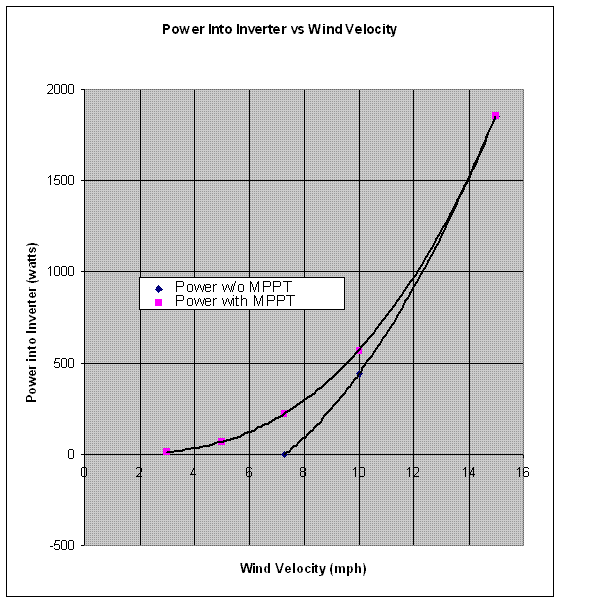Shown are two plots; one with mppt, the other without. Notice the increase in power delivered to the inverter with mppt. This is especially significant for South Central PA with average wind velocities less than 8 mph.

Background:
The design requirements that I imposed on this circuit are listed below:

1. The circuit should operate in parallel with the main rectifier bank.

2. The circuit should load the windmill generator so the blades operate near their design tip speed ratio (TSR) for wind velocities between 3 miles per hour (mph) up to the design point wind velocity where the blade TSR naturally occurs. This design point velocity for my 20 foot diameter windmill and generator (shown here: http://www.fieldlines.com/story/2009/3/2/171331/4786) is about 18 mph.

3. The circuit should cut in at wind velocities approaching 3 mph and cut out at wind velocities exceeding the design point (18 mph). This is done to avoid overloading circuit components. Besides, there is little energy available at wind speeds under 3 mph. At wind speeds over 18 mph, I am starting to shed some power through furling and stall and don’t need MPPT.

4. Use analog components that are readily available at Radio Shack or on eBay.

Approach:
There are three basic sub circuits that make up the MPPT system. The block diagram for the MPPT circuits is shown below: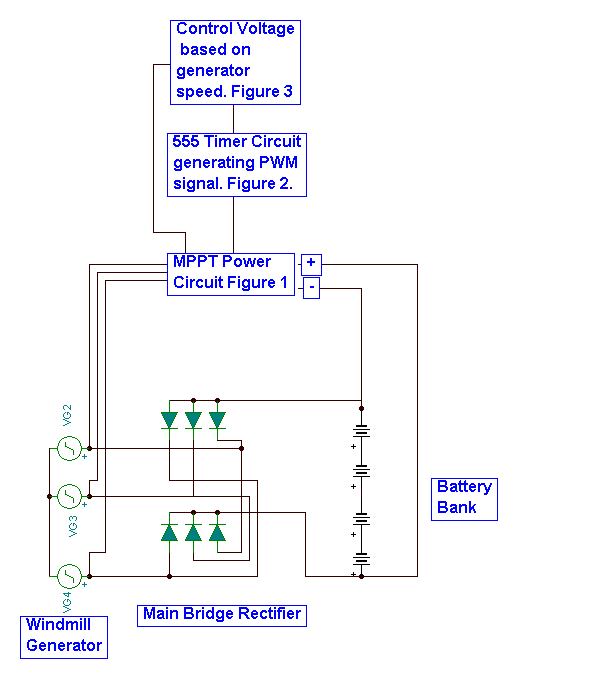These sub circuits, are:
1. Power shown in Figure 1.This circuit takes power from the generator and transfers it to the battery bank. It receives a pulse width modulated (PWM) signal from a 555 timer circuit that is used to control the voltage at the bridge rectifier “DC+” terminal. Controlling this voltage sets the power transferred to the battery and regulates the load on the generator to assure MPPT.

2. The 555 timer circuit is shown in Figure 2. It generates the PWM signal as a function of a voltage proportional to generator speed.

3. The circuit that generates the pulse width control voltage proportional to generator speed is shown in Figure 3. This voltage is also tailored to cause cut in and cut out the MPPT system.

The details of these circuits are shown, below:
1. Power (Figure 1)
The power portion of the circuit is shown here: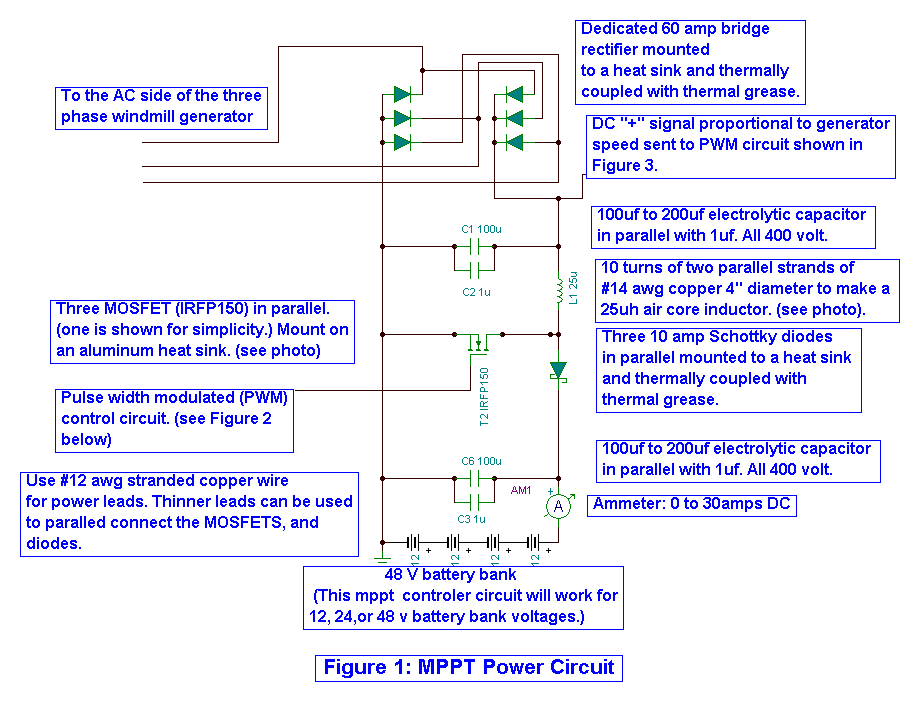It is a relatively standard boost converter design whose purpose is to load the generator so it operates at the design TSR for a wide range of wind speeds. This is achieved by controlling the DC “+” voltage at the bridge rectifier.

2. PWM Circuit (Figure 2)

The PWM circuit uses a 555 timer circuit set up to create a 10khz pulse modulated with a voltage signal ranging from about 4 volts (0% pulse width) to about 8 volts (100% pulse width).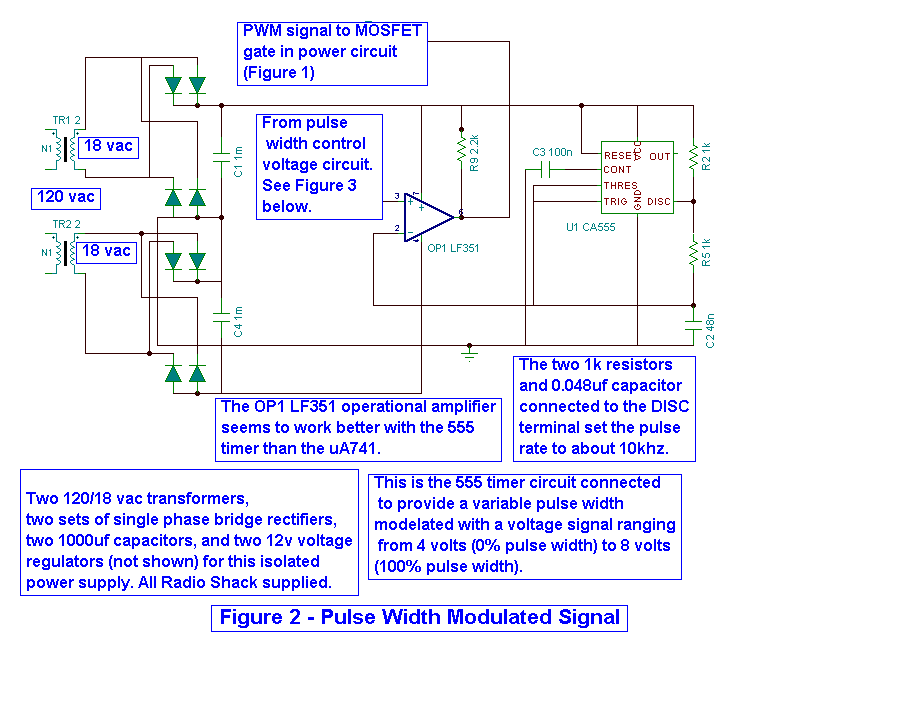3. Control Voltage (Figure 3)
The pulse width control voltage portion of the circuit is the brains of the MPPT system. The circuit is shown below: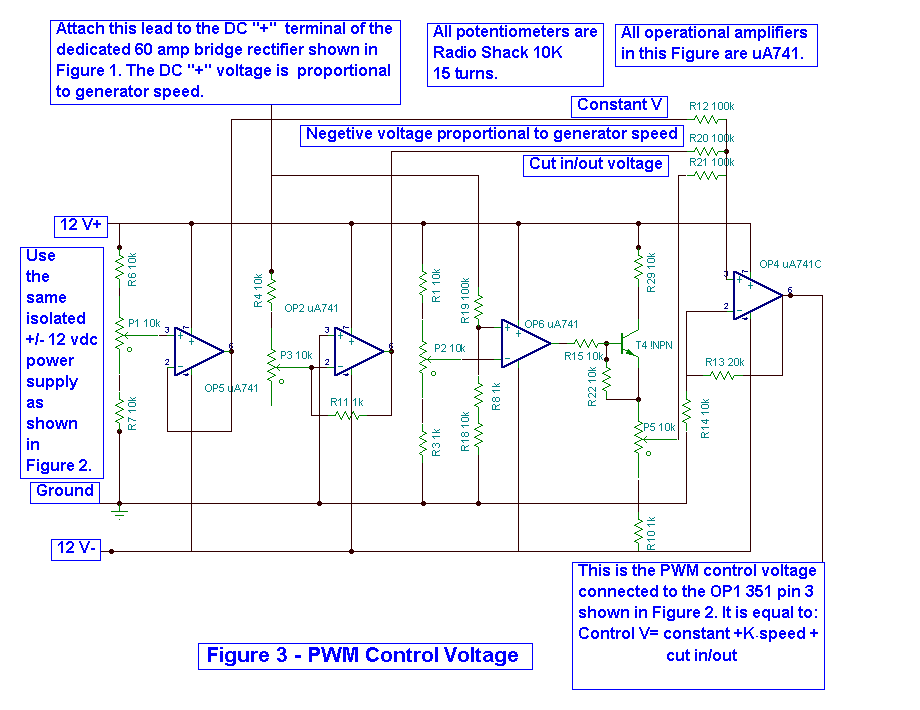D: Performance:
One question that could be asked, is: “How does this circuit provide MPPT through out the selected wind speed range?” The following is my answer:

Optimally loaded wind turbines run at about half their unloaded rpm at their design TSR. This can be seen in measured data such as that provided by Princeton University for their sail wing design. Princeton’s data are shown below: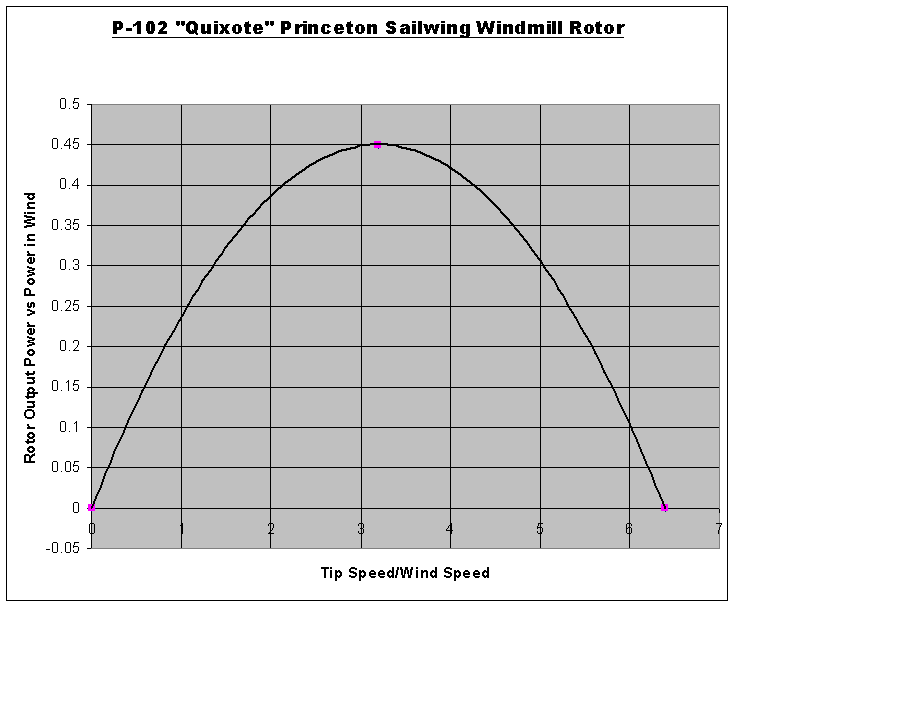You can see that peak power occurs at roughly one half the unloaded TSR; For example, my 20’ diameter windmill was designed for a TSR=7. Unloaded, it will run at a TSR approximately = to 14.

Our windmills charging a battery bank without MPPT will operate at the design TSR at only one wind velocity. For example, my 20 foot diameter windmill starts making power (“cuts in”) at 7.3 mph with a TSR ~14. Its design TSR is 7. As the wind velocity increases, the operating TSR reduces from 14 to 7 as the wind velocity increases from 7.3 to 18 mph. Above 18 mph, the TSR moves from 7 toward 0. This all occurs with a battery voltage ~ 52 volts.

There are ways to load the wind turbine so it operates at its design TSR for all wind speeds above cut-in. One way involves changing the “apparent” battery voltage in proportion to wind velocity. The boost converter shown in Figure 1 does this by controlling the voltage (Vr) at the “DC+” terminal of the bridge rectifier. The output of the boost converter is clamped at the battery voltage.

Vr is related to the design TSR, as shown in the following equation:

Vr = generator voltage * 3^^.5 at design TSR - I * R drop in generator and line - drop across bridge rectifier

I solved this equation for my windmill and have plotted the results below: (Don’t worry; you won’t have to calculate this for yours, if you don’t want to. There is a simpler way.) I’m showing this for those interested in the physics.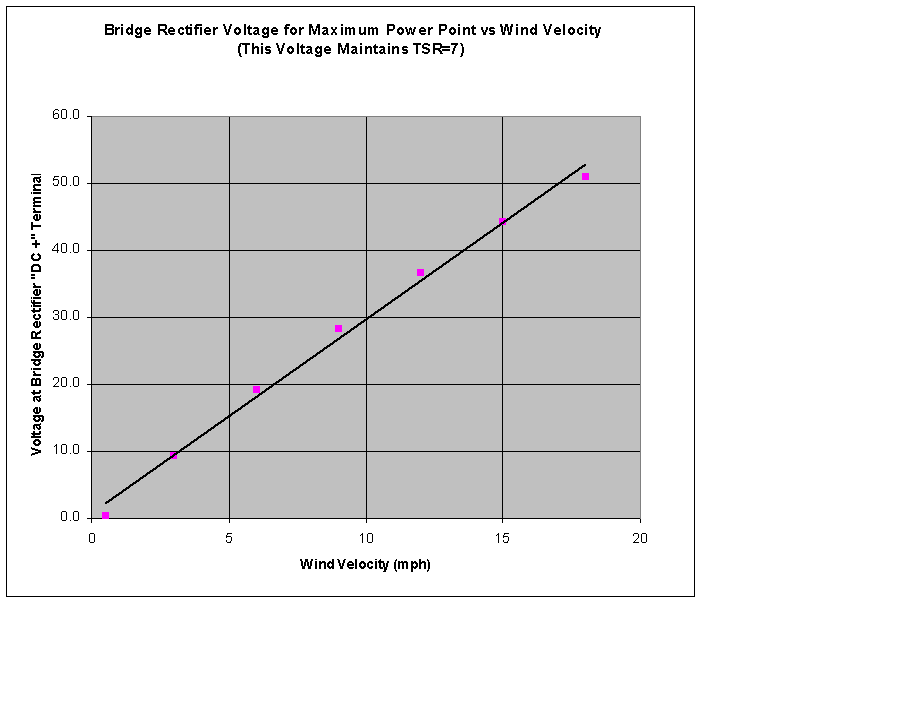Notice the almost linear relationship between Vr and wind velocity. This occurs because the IR voltage drop through the generator and line resistances are small compared to generator voltage. This simplifies the controls. It also, makes Vr proportional to generator speed, especially at low wind velocities where generator current is low.

The MPPT circuit potentiometers are adjusted to achieve the above Vr, as shown in the System Alignment section, below. In effect, it uses the Boost circuit to provide an apparent battery voltage as a function of wind velocity.

The 555 timer circuit of Figure 2 provides a PWM signal to the MOSFET gates in Figure 1. The pulse duty cycle varies in a way to provide Vr, as a function of wind velocity. The relationship that works for my 20’ is shown below: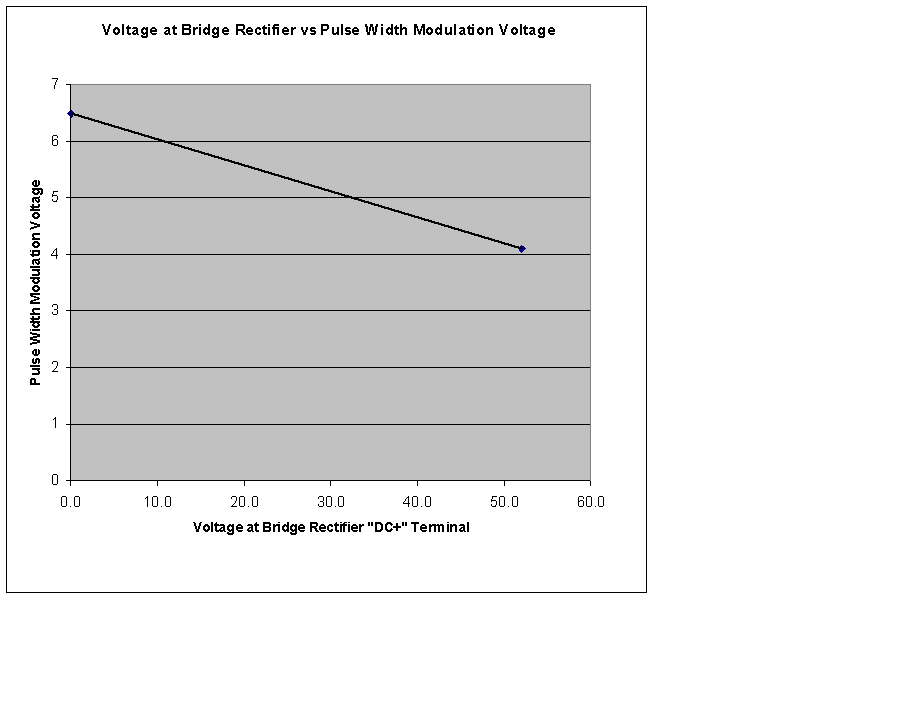This relationship can be achieved by analysis or by using the system alignment procedure covered below. The system alignment procedure is a better choice than analyses because it accounts for “as built” imperfections in the system.

System Alignment

The potentiometers in the above circuit need to be adjusted so the system does maximum power point tracking (MPPT). The following procedure works for me:

A: Preliminary Adjustment (can be done on the bench)
1. Connect all three subsystems together as shown in Figures 1, 2, and 3 with the exception of the three leads going to the three phase windmill generator. Plug in the power cord to the auxiliary +/-12v power supply.
2. Confirm the presence of power on the +12v buss and -12v buss.
3. Adjust potentiometer P2 to achieve the DC cut in voltage/10 that will occur during low (~3mph) wind speeds. I recommend about 20% of your battery bank voltage for starters. For my 48 system this corresponds to about 10.5v/10=1.05v. This cut in set point voltage can be measured on the negative input terminal of OP6.
4. Remove the lead from the DC “+” terminal and temporarily connect it to the battery bank “+” terminal.
5. Adjust potentiometer P5 to achieve 0.00v on the wiper portion of P5. This is also the “cut in/out voltage” at R21 that occurs at generator speeds above the cut in set point voltage of Step 3, above.
6. Adjust potentiometer P1 to achieve a preliminary voltage of about 6.5v at R12. This voltage is constant independent of generator speed.
7. Adjust potentiometer P3 to achieve a preliminary voltage of about -2.5v at R20. This voltage varies in proportion to generator speed. The PWM control voltage (OP4, pin 6, Figure 3) = Constant V (R12)-Kgen speed (R20) should = 6.5-2.5= 4.00 volts
8. Return the lead back to the DC”+” terminal.
9. Unplug the auxiliary power supply.

B: Final adjustment of P1. (should be done on the windmill) Make this adjustment during constant wind velocity conditions between the cut in set point (Step 3) and normal cut in of the main power path. For my system this corresponds to wind velocities over 3mph and under about 7mph.
1. Connect the three phase leads to the generator output upstream of the main rectifier bank.
2. Plug in the auxiliary power supply.
3. The ammeter should indicate current to the battery bank if everything is working properly and if the generator DC “+” voltage/10 exceeds the cut in set point voltage of Step 3, Preliminary, above.
4. Adjust the system to provide operation at the design TSR, by confirming that the generator speed (DC “+” voltage) doubles when the auxiliary power supply is unplugged (no MPPT) during a nearly constant wind condition. Check this in steady wind conditions at velocities under main cut in, but exceeding the cut in voltage set point in Step 3. If the generator’s speed more than doubles, adjust P1 to lower the voltage at R12. If the speed doesn’t double, raise the voltage. Warning: Do not exceed 7.5 v at R12 or you will risk turning the MOSFETS on 100% without pulsing. This will cause a dead short and possibly smoke them.

C: Final adjustment of P3: Remove the lead from the rectifier DC + terminal and attach it to the battery + terminal. Adjust P3 until the voltage at R20 satisfies the following equation:

PWM control voltage (OP4, pin 6, Figure 3) = Constant V (R12) - Kgen speed (R20)
= 4.00 volts

The 4.00v PWM control voltage will be reached when Vr = Vb and will result in a PWM duty cycle ~ 0 .
Return the lead to the rectifier DC+ terminal.

Repeat Sections B and C, several times to assure accuracy. Also, I weekly check the settings made by each of the potentiometers to help assure drift has not occurred. This check should continue until you gain confidence in the system.

You now should have an operating MPPT system.

BobS